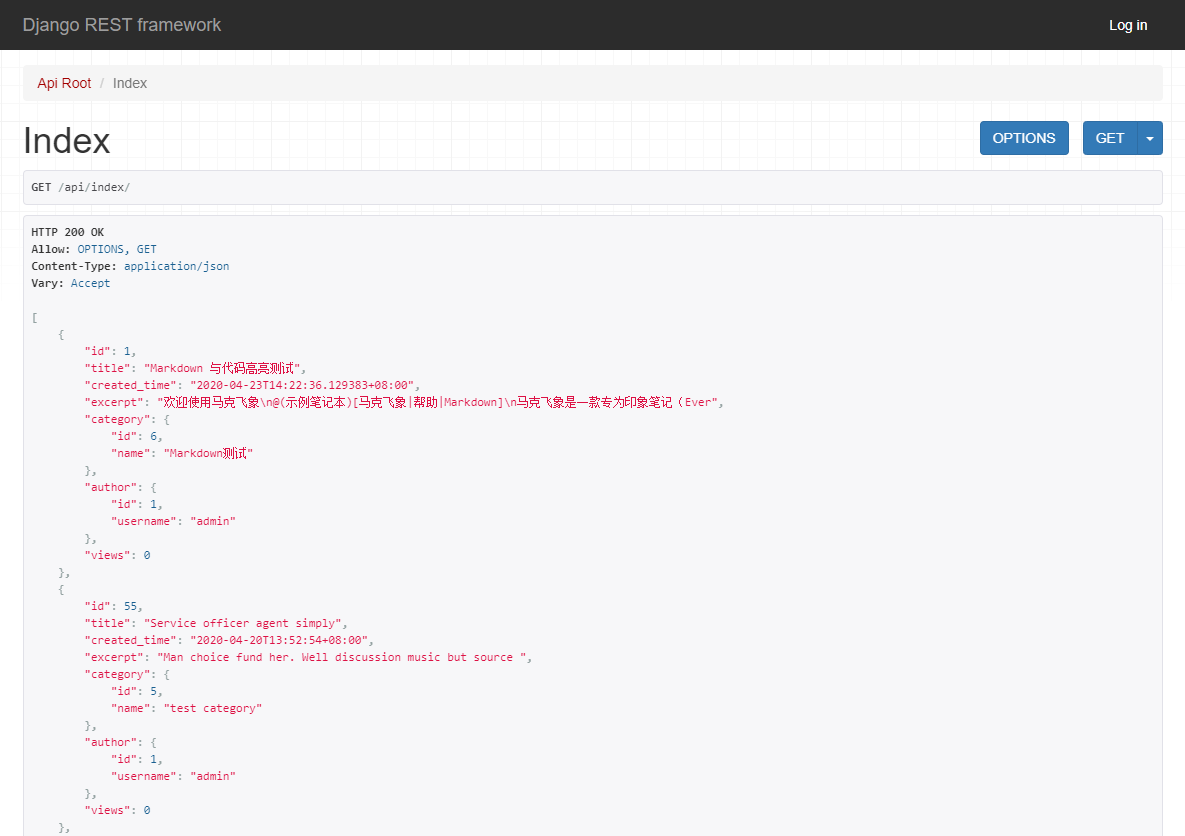# 实现博客首页文章列表 API

``````from django.shortcuts import render
from .models import Post

def index(request):
post_list = Post.objects.all().order_by('-created_time')
return render(request, 'blog/index.html', context={'post_list': post_list})
``````

``````from rest_framework.decorators import api_view
from rest_framework.response import Response
from rest_framework import status

from .models import Post
from .serializers import PostListSerializer

@api_view(http_method_names=["GET"])
def index(request):
post_list = Post.objects.all().order_by('-created_time')
serializer = PostListSerializer(post_list, many=True)
return Response(serializer.data, status=status.200)
``````

200：通常表示请求成功。

201：表示资源创建成功。

400：表示客户端请求错误。

401：没有提供身份认证信息

403：没有操作权限

404 ：访问的资源不存在

405：不支持的 HTTP 请求方法

500：服务器内部错误

HTTP 请求和响应过程，django-rest-framework 已经帮我们处理。但是资源的序列化，框架是无法自动化完成的，框架提供了基本的序列化器，我们需要自定义序列化逻辑。所以，让我们来定义 `PostListSerializer` 序列化器，用它来序列化文章列表。

``````from datetime import datetime

class Comment(object):
def __init__(self, email, content, created=None):
self.email = email
self.content = content
self.created = created or datetime.now()

comment = Comment(email='leila@example.com', content='foo bar')
``````

``````from rest_framework import serializers

email = serializers.EmailField()
content = serializers.CharField(max_length=200)
created = serializers.DateTimeField()
``````

``````>>> serializer = CommentSerializer(comment)
>>> serializer.data
# 输出：
{'email': 'leila@example.com', 'content': 'foo bar', 'created': '2016-01-27T15:17:10.375877'}
``````

``````from rest_framework import serializers
from .models import Post

class PostListSerializer(serializers.ModelSerializer):
category = CategorySerializer()
author = UserSerializer()

class Meta:
model = Post
fields = [
'id',
'title',
'created_time',
'excerpt',
'category',
'author',
'views',
]
``````

``````class PostListSerializer():
id = IntegerField(label='ID', read_only=True)
title = CharField(label='标题', max_length=70)
created_time = DateTimeField(label='创建时间', required=False)
excerpt = CharField(allow_blank=True, label='摘要', max_length=200, required=False)
category = CategorySerializer()
author = UserSerializer()
``````

``````from django.contrib.auth.models import User
from .models import Category, Post

class CategorySerializer(serializers.ModelSerializer):
class Meta:
model = Category
fields = [
'id',
'name',
]

class UserSerializer(serializers.ModelSerializer):
class Meta:
model = User
fields = [
'id',
]

class PostListSerializer(serializers.ModelSerializer):
# ...
``````

``````@api_view(http_method_names=["GET"])
def index(request):
post_list = Post.objects.all().order_by('-created_time')
serializer = PostListSerializer(post_list, many=True)
return Response(serializer.data, status=status.HTTP_200_OK)
``````

`api_view` 装饰器传入 `http_method_names` 参数指定允许访问该 API 视图的 HTTP 方法。

``````path('api/index/', views.index)
``````1. 从数据库取数据
2. 构造序列化器并将取出的数据序列化
3. 返回响应

-- EOF --